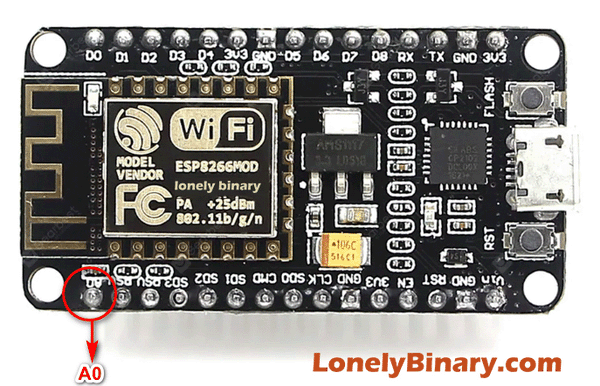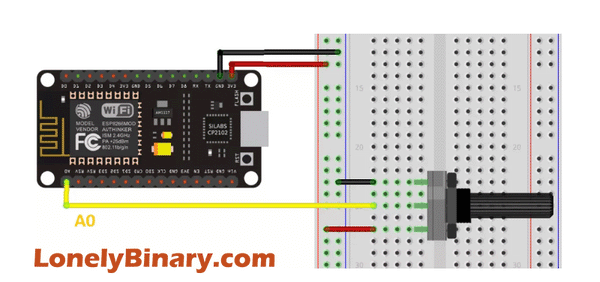ESP8266 have ONE ADC pin that is easily accessible. This means that those ESP8266 boards can read analog signals. In this tutorial we’ll show you how to use analog reading with the ESP8266 using Arduino IDE.

• 10-bit resolution, which means you’ll get values between 0 and 1024.
• Voltage range in ESP8266 development boards: 0 to 3.3V.
• Pin:  A0DiagramArduino Script

```const int analogInPin = A0;  // ESP8266 Analog Pin ADC0 = A0

int sensorValue = 0;  // value read from the pot
int outputValue = 0;  // value to output to a PWM pin

void setup() {
// initialize serial communication at 115200
Serial.begin(115200);
}

void loop() {

// map it to the range of the PWM out
outputValue = map(sensorValue, 0, 1024, 0, 255);

// print the readings in the Serial Monitor
Serial.print("sensor = ");
Serial.print(sensorValue);
Serial.print("\t output = ");
Serial.println(outputValue);

delay(1000);
}```